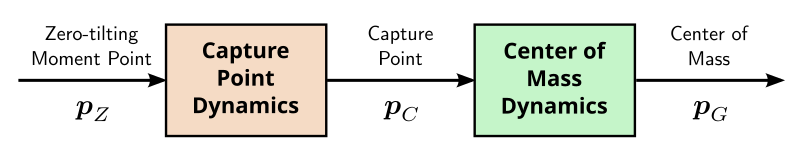# Capture point

The capture point is a characteristic point of the linear inverted pendulum model. It was coined by Pratt et al. (2006) to address a question of push recovery: where should the robot step (instantaneously) to eliminate linear momentum $$m \bfpd_G$$ and come (asymptotically) to a stop?

## Derivation

Let us start from the equation of motion of the system:

\begin{equation*} \bfpdd_G = \omega^2 (\bfp_G - \bfp_Z) \end{equation*}

We assume that the robot steps instantly at time $$t=0$$ and maintains its ZMP at a constant location in its new foothold, so that $$\bfp_Z$$ is stationary. Given that the natural frequency $$\omega$$ of the pendulum is also a constant, we can solve this second-order linear differential equation as:

\begin{equation*} \bfp_G(t) = \displaystyle \bfp_Z + \frac{e^{\omega t}}{2} \left[\bfp_G(0) + \frac{\bfpd_G(0)}{\omega} - \bfp_Z\right] + \frac{e^{-\omega t}}{2} \left[\bfp_G(0) - \frac{\bfpd_G(0)}{\omega} - \bfp_Z\right] \end{equation*}

This function is the sum of a stationary term $$\bfp_Z$$, a convergent term factored by $$e^{-\omega t}$$ that vanishes as $$t \to \infty$$, and a term factored by $$e^{\omega t}$$ that diverges as $$t \to \infty$$. Let us define the capture point as:

\begin{equation*} \bfp_C \defeq \bfp_G + \frac{\bfpd_G}{\omega} \end{equation*}

The divergent term in $$\bfp_G(t)$$ is then $$e^{\omega t}/2 (\bfp_C(0) - \bfp_Z)$$. In particular, the only way for the center of mass trajectory to be bounded is for the stationary ZMP to be equal to the instantaneous capture point:

\begin{equation*} \bfp_Z = \bfp_C(0) \ \Longrightarrow \ \bfp_G(t) \underset{t \to \infty}{\longrightarrow} \bfp_C(0) \end{equation*}

We can thus interpret the capture point as a point where the robot should step (shift its ZMP) in order to come (asymptotically) to a stop.

## Discussion

The capture point is a divergent component of motion of the linear inverted pendulum. Shifting the ZMP to the capture point prevents divergence from the unstable dynamics of the model, but does not control the other (stable) component. In effect, the system comes to a stop following its natural dynamics:

\begin{equation*} \bfpd_G = \omega (\bfp_C - \bfp_G) \end{equation*}

This phenomenon is noticable in balance controllers based on capture point feedback such as Englsberger et al. (2011) and Morisawa et al. (2012). Take the robot standing, push it in a given direction and sustain your push, then suddenly release it: the robot will come back to its reference standing position following its natural dynamics (which only depend on $$\omega$$, i.e. gravity and the height of the center of mass), regardless of the values of the various feedback gains used in the balance controller. You can for instance test this behavior in dynamic simulations with the lipm_walking_controller.

This behavior highlights how balance controllers based on capture-point feedback are not trying to come to a stop as fast as possible. Rather, they focus on preventing divergence, and leverage passive dynamics to absorb undesired linear momentum. When using linear feedback, Sugihara (2009) showed that this approach maximizes the basin of attraction of the resulting controller.

## Boundedness condition

The derivation above can be generalized to the case where $$\bfp_Z(t)$$ is time-varying rather than time-invariant. Consider the equation of motion split as follows into divergent and convergent components:

\begin{equation*} \begin{array}{rcl} \bfpd_C & = & \omega (\bfp_C - \bfp_Z) \\ \bfpd_G & = & \omega (\bfp_C - \bfp_G) \end{array} \end{equation*}

The capture point diverges away from the ZMP while the center of mass is attracted to the capture point:As the center-of-mass dynamics are convergent, the system diverges if and only if its capture point diverges. We can therefore focus on the capture point dynamics alone.

The solution to a first-order linear time-varying differential equation is:

\begin{equation*} \dot{\bfy}(t) - a(t) \bfy(t) = \bfb(t) \ \Longrightarrow \ \bfy(t) = e^{A(t)} \left(\bfy(0) + \int_{\tau=0}^t \bfb(\tau) e^{-A(\tau)} {\rm d} \tau \right) \end{equation*}

where $$A$$ is the antiderivative of $$a$$ such that $$A(0)=0$$. Applied to capture point dynamics, this formula becomes:

\begin{equation*} \bfp_C(t) = e^{\omega t} \left(\bfp_C(0) - \omega \int_{\tau=0}^t \bfp_Z(t) e^{-\omega \tau} {\rm d}\tau\right) \end{equation*}

We can check how, in the previous case where $$\bfp_Z$$ is stationary, this formula becomes:

\begin{equation*} \bfp_C(t) = \bfp_Z + e^{\omega t} (\bfp_C(0) - \bfp_Z) \end{equation*}

The capture point trajectory is then bounded if and only if $$\bfp_Z = \bfp_C(0)$$, which is indeed the result we obtained above. In the general case, the capture point stays bounded if and only if:

\begin{equation*} \bfp_C(0) = \omega \int_{\tau=0}^t \bfp_Z(t) e^{-\omega \tau} {\rm d}\tau \end{equation*}

This condition was coined boundedness condition by Lanari et al. (2014). It relates future system inputs to the present state, and characterizes the subset of these inputs that will actually stabilize the system in the long run. The boundedness condition is, for instance, a core component of the walking pattern generator from Scianca et al. (2019). It can also be applied to more general reduced models such as the variable-height inverted pendulum.

## To go further

The notion of divergent component of motion behind the capture point reaches beyond the linear inverted pendulum model. Check it out for extensions to more advanced balance control.

Pages of this website are under the CC-BY 4.0 license.